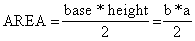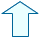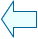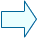GEOMETRIC SHAPES ON THE PLANE_2 Section: Geometry

 1. THE AREA OF A TRIANGLE (starting with the area of a rectangle) The next few windows are going to help us work out the formula for finding the area of a triangle:We are going to use a technique that we have already used a lot in this unit, which is: use what we already know (the formula of a shape) to help us work out what we don't know (a formula of a different shape). Here, we are going to work out the formula of a triangle from that of a parallelogram. Remember that we worked out the formula of a parallelogram from that of a rectangle.The window below shows us how to use the formula of a rectangle to work out that of a triangle. We are going to look at this before looking at a more commonly used example. 1.- Look at how triangle ABC is divided into two triangles by height h. 2.- Use the "show" control button to see how for each triangle there is another darker-coloured triangle which is the same size. 3.- Note that therefore, the area of a rectangle is twice the area of a triangle. 4.- Use the rectangle we constructed earlier to work out the formula  to find the area of a triangle. You should see that we just need to divide the area of a rectangle in half.
 5.- Although the construction above is straight forward, there is one drawback: The construction cannot be made if the projection of point B falls outside the segment AC. Try it yourself and see.

2. THE AREA OF A TRIANGLE (in general)

We are going to work out a general formula to find the area of any triangle. We are going to do this step by step, by seeing how the triangle relates to the parallelogram, as shown in the window below.

1.- Use the next_step control button and answer the questions that appear in the window.

 The Init button will restore the initial values.

3. THE AREA OF TRIANGLES WITH THE SAME HEIGHT

In the following window we are going to see what happens if we drag point B and then point A.

1.- Drag point B and note that new triangles are formed. Then, try dragging point A. What do all these triangles have in common? Is the base of each one the same? Is the height of each one the same? Can you therefore use the previous formula to work out if the area of each of the triangles is the same?

 If you click on the exercise button you should be able to answer the question that appears. In order to do so, move the original triangle by dragging the points as necessary.

2.- Is it true to say that the perimeter of all the triangles which are formed by dragging point B along the straight line are equal?Agustín Muñoz NúñezSpanish Ministry of Education. Year 2001